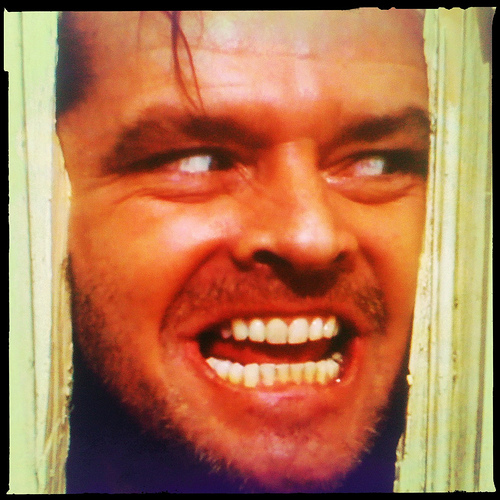# Really Irrational Equations

Algebra Level 5

There exist a real number $p$such that the following system of equations

$\dfrac { 1 }{ \sqrt { 1+2{ x }^{ 2 } } } +\dfrac { 1 }{ \sqrt { 1+2{ y }^{ 2 } } } =\dfrac { 2 }{ \sqrt { 1+2xy } }$

$x\sqrt { x(1-2x) } +\sqrt { y(1-2y) } =p$

has only one real value $x$ as a root, which can be expressed as

$x=\dfrac { a+\sqrt { b } }{ c }$

where $a, b, c$ are all non-zero integers and $b$ is square-free. Find $a+b+c$

Details and Assumptions:

• There's no typo in this question.

See Victor Loh's Irrational Equations

×